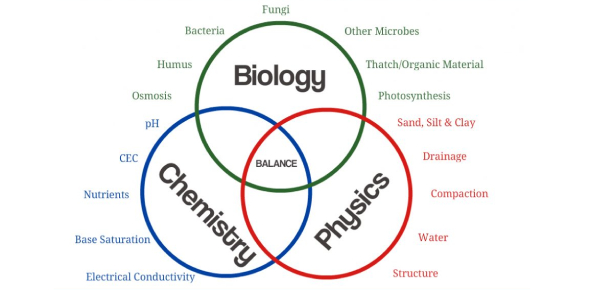# Science Quiz For Class 11 And 12

10 Questions | Attempts: 45993
ShareSettingsAre you ready for an ultimate science challenge test? That’s right! Given below is a Science quiz for class 11 and 12 students. In this test, we’ll be looking at the core three topics that constitute scientific study; biology, physics, and chemistry, which give us so much of the information we need to understand life and the universe around us. Do you think you can score well on this test? Let's see how you perform.

• 1.
A person sitting in a moving bus suddenly moves forward when the bus suddenly stops?
• A.

Due to inertia

• B.

Due to brakes

• C.

Due to negative acceleration

• D.

Due to force exerted by the machine

• E.

None of these

• 2.
The person sitting in a moving car has _________energy.
• A.

Kinetic energy

• B.

Potential energy

• C.

Both kinetic and potential energy

• D.

Elastic potential energy

• E.

Gravitational potential energy

• 3.
Why in hitting the carpet with stick mud flies away?
• A.

Due to friction

• B.

Due to inertia

• C.

None of these

• D.

Due to force of stick

• E.

Both A and B

• 4.
Why are transition elements called so?
• A.

Variable valency

• B.

Having different colors

• C.

Constant valency

• D.

None of these

• E.

Both A and B

• 5.
Why is it challenging to prepare a supersaturated solution?
• A.

None of these

• B.

Because it cant be prepared at room temperature

• C.

Solute particles cant be homogenised

• D.

Constant heat pressure

• E.

Oxygen in air

• 6.
Is electrolysis same to ionization? If no, why?
• A.

Yes!

• B.

Electrolysis can't be done without ionization

• C.

It can be done

• D.

Matter splits up into its ions

• E.

None of these

• 7.
Why on hitting the ball with the center of the bat more effective short is played?
• A.

Fx + fy= o

• B.

Center of gravity remained within its base

• C.

It produces more torque

• D.

Both A and B

• E.

None of these

• 8.
What are Jacobson's organs?
• A.

Two pits present on palate on snake

• B.

Nostrils

• C.

Organs present in note

• D.

Tachea

• E.

None of these

• 9.
Each rank of classification is called:
• A.

Texon

• B.

Evolutionary taxonomy

• C.

Homology

• D.

Analogy

• E.

None of these

• 10.
Enthalapy of products of exothernic reaction is_________.
• A.

Less

• B.

More

• C.

Sometimes less and sometimes more

• D.

Equal

• E.

None of these

## Related TopicsBack to top
×

Wait!
Here's an interesting quiz for you.